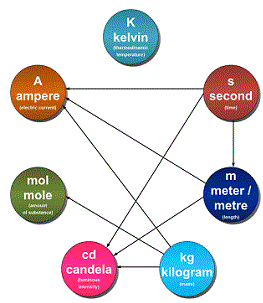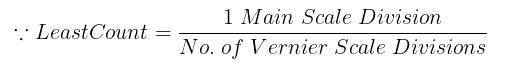Courses

# Test: Units & Measurements- 2

## 30 Questions MCQ Test Physics Class 11 | Test: Units & Measurements- 2

Description
This mock test of Test: Units & Measurements- 2 for JEE helps you for every JEE entrance exam. This contains 30 Multiple Choice Questions for JEE Test: Units & Measurements- 2 (mcq) to study with solutions a complete question bank. The solved questions answers in this Test: Units & Measurements- 2 quiz give you a good mix of easy questions and tough questions. JEE students definitely take this Test: Units & Measurements- 2 exercise for a better result in the exam. You can find other Test: Units & Measurements- 2 extra questions, long questions & short questions for JEE on EduRev as well by searching above.
QUESTION: 1

### Physical quantities are:

Solution:
• A physical quantity is a physical property of a phenomenon, body, or substance, that can be quantified by measurement.
• A physical quantity can be expressed as the combination of a magnitude expressed by a number – usually a real number – and a unit.
• All these given above can be expressed as explained so these are physical quantity.
QUESTION: 2

### If radian correction is not considered in specific heat measurement. The measured value of specific heat will be:

Solution:

If radian correction is not considered in specific heat measurement. The measured value of specific heat will be more than its actual value.

QUESTION: 3

### A new unit of length is chosen such that the speed of light in vacuum is unity. What is the distance between the Sun and the Earth in terms of the new unit if light takes 8 min and 20 s to cover this distance?

Solution:

► Time can be converted as t = (8 x 60) + 20s = 500 seconds
► Now speed given= 1 unit
We know speed = Distance / Time
which implies distance = Speed x Time
Hence, the distance becomes 1 x 500 = 500 units.

QUESTION: 4

The length, breadth and thickness of a rectangular sheet of metal are 4.234 m, 1.005 m, and 2.01 cm respectively. Give the volume of the sheet to correct significant figures.

Solution:

Length , l = 4,234 m
Breadth, b = 1.005 m
Thickness , t = 2.01 x 10-2 m
Volume = l x b x t
⇒ V = 4.234 x 1.005 x 0.0201 = 0.0855289 = 0.0855 m3 (significant figure = 3)

QUESTION: 5

The number of significant digits in 2,076 is:

Solution:

There are three rules for determining how many significant figures are in a number:

• Non-zero digits are always significant.
• Any zeros between two significant digits are significant.
• A final zero or trailing zeros in the decimal portion ONLY are significant.

Keeping these rules in mind, we can say that there are 4 significant digits.

QUESTION: 6

Measurement of a physical quantity is essentially the:

Solution:

The Measurement of a given quantity is essentially an act or result of comparison between a quantity whose magnitude (amount) is unknown, with a similar quantity whose magnitude (amount) is known, the latter quantity being called a Standard.

QUESTION: 7

The relative error in a physical quantity raised to the power k is:

Solution:

The relative error in a physical quantity raised to the power k is the k times the relative error in the individual quantity.

Suppose Z = A2,
Then,
ΔZ/Z = (ΔA/A) + (ΔA/A) = 2 (ΔA/A).
Hence, the relative error in A2 is two times the error in A.

QUESTION: 8

The number of significant digits in 0.007 is:

Solution:

There are three rules on determining how many significant figures are in a number:

• Non-zero digits are always significant.
• Any zeros between two significant digits are significant.
• A final zero or trailing zeros in the decimal portion ONLY are significant.

So, keeping these rules in mind, there are 1 significant digit.

QUESTION: 9

The number of significant digits in 48,923 is:

Solution:

There are three rules on determining how many significant figures are in a number:

• Non-zero digits are always significant.
• Any zeros between two significant digits are significant.
• A final zero or trailing zeros in the decimal portion ONLY are significant.

Keeping these rules in mind, we can say that there are 5 significant digits.

QUESTION: 10

Unit for a fundamental physical quantity is:

Solution:

Unit is the reference used as the standard measurement of a physical quantity.

• The unit in which the fundamental quantities are measured are called fundamental unit.
• Units used to measure derived quantities are called derived units.
QUESTION: 11

The significant figures of a number ______.

Solution:

Zeros occurring between non zero digits also contribute to the number accuracy and thus all the digits that contribute towards the accuracy of the number are considered significant.

QUESTION: 12

The mass of a box measured by a grocer’s balance is 2.300 kg. Two gold pieces of masses 20.15 g and 20.17 g are added to the box. What is the difference in the masses of the pieces to correct significant figures?

Solution:

Difference in masses = 20.17 – 20.15 = 0.02 g
In subtraction, the final result should retain as many decimal places as there are in the number with the least decimal places.

QUESTION: 13

Horse power is unit of:

Solution:
• The horsepower (hp) is a unit in the foot-pound-second ( fps ) or English system, sometimes used to express the rate at which mechanical energy is expended.
• It was originally defined as 550 foot-pounds per second (ft-lb/s).
• A power level of 1 hp is approximately equivalent to 746 watt.
QUESTION: 14

The number of significant digits in 8.1000 is:

Solution:

There are three rules on determining how many significant figures are in a number:

• Non-zero digits are always significant.
• Any zeros between two significant digits are significant.
• A final zero or trailing zeros in the decimal portion ONLY are significant.

Keeping these rules in mind, we can say that there are 5 significant digits.

QUESTION: 15

In SI system the fundamental units are:

Solution:

The SI base units and their physical quantities are the metre for measurement of length, the kilogram for mass, the second for time, the ampere for electric current, the kelvin for temperature, the candela for luminous intensity, and the mole for amount of substance.QUESTION: 16

The dimensions of a physical quantity are:

Solution:

The dimension of the units of a derived physical quantity may be defined as the number of times the fundamental units of mass, length and time appear in the physical quantity.

The expression for velocity obtained above is said to be its dimensional formula. Thus, the dimensional formula for velocity is [M0L1T​​​​​​-1].

QUESTION: 17

The significant digits in 0.000532 are:

Solution:

There are three rules on determining how many significant figures are in a number:

• Non-zero digits are always significant.
• Any zeros between two significant digits are significant.
• A final zero or trailing zeros in the decimal portion ONLY are significant.

Keeping these rules in mind, we can say that only 5,3,2 are significant digits.

QUESTION: 18

One kilometer is equal to how many miles?

Solution:

for an approximate result, divide the length value by 1.609

► One kilometer is equals to 0.62 miles.

QUESTION: 19

Population of a town is reported as 157,900 . Which of the following statements correct?

Solution:

157900  implies that the population is believed to be within the range of about 157850 to about 157950.

In other words, the population is 157900 ± 50. The “plus-or-minus 50” appended to this number means that we consider the absolute uncertainty of the population measurement to be 50 – (–50) = 100.

We can also say that the relative uncertainty is 100/157900, which we can also express as 1 part in 1579, or 1/1579 = 0.000633, or about 0.06 percent.

QUESTION: 20

Derived units:

Solution:

Derived units are units which may be expressed in terms of base units by means of mathematical symbols of multiplication and division.
Example: the SI derived unit of area is the square metre (m​​​​​​2), and the SI derived unit of density is the kilogram per cubic metre (kg/m3 or kg m​​​​​​-3).

The names of SI units are written in lowercase.

QUESTION: 21

According to the principle of homogeneity of dimensions:

Solution:

It would be more exact to say that "Quantities with the same units can be added and subtracted with no problem."

Quantities with the same dimensions can often be added and subtracted as long as the units are correctly converted.

QUESTION: 22

There are 20 divisions in 4 cm of the main scale. The vernier scale has 10 divisions. The least count of the instrument is:

Solution:

There are 20 divisions in 4 cm of main scale
⇒ 1 cm of main scale will have 20/4 = 5 divisions

Thus,  1 MSD  = 1/5 = 0.2 cm Vernier scale has 10 divisionsThus, L.C. = 0.2/10 = 0.02 cm

QUESTION: 23

Joule is the SI unit of:

Solution:

The joule (symbol J), is a derived unit of energy in the International System of Units.

It is equal to the energy transferred to (or work done on) an object when a force of one newton acts on that object in the direction of its motion through a distance of one metre (1 newton metre or N⋅m).

QUESTION: 24

If an equation is dimensionally consistent:

Solution:

The principle of homogeneity of dimensions states that “For an equation to be dimensionally correct, the dimensions of each term on LHS must be equal to the dimensions of each term on RHS.”

QUESTION: 25

An object has a length of 0.42 cm. Which of the following statements correct?

Solution:

As no indication of its precision is given. In this case, all we have to go on is the number of digits contained in the data.

Thus the quantity “0.42 cm” is specified to 0.01 unit in 0.42, or one part in 42.

The implied relative uncertainty in this figure is 1/42, or about 2%.

QUESTION: 26

The least count of any measuring equipment is:

Solution:

The smallest value that can be measured by the measuring instrument is called its least count.

Measured values are good only up to this value. The least count error is the error associated with the resolution of the instrument.

QUESTION: 27

Dimensional analysis can be used to:

Solution:

Dimensional Analysis (also called Factor-Label Method or the Unit Factor Method) is a problem-solving method that uses the fact that any number or expression can be multiplied by one without changing its value. It is a useful technique.

Another use of dimensional analysis is in checking the correctness of an equation which you have derived after some algebraic manipulation. Even a minor error in algebra can be detected because it will often result in an equation which is dimensionally incorrect.

QUESTION: 28

The result of rounding off 34.216 to 3 digits is:

Solution:

The rules for rounding off are following:

• If the first non-significant digit is less than 5, then the least significant digit remains unchanged.
• If the first non-significant digit is greater than 5, the least significant digit is incremented by 1.
• If the first non-significant digit is 5, the least significant digit can either be incremented or left unchanged.
• All non-significant digits are removed.

So rounding off 34.216 upto 4 digits is 34.22 and upto 3 digits is 34.2

QUESTION: 29

Resolution is:

Solution:

Resolution is the smallest amount of input signal change that the instrument can detect reliably. This term is determined by the instrument noise (either circuit or quantization noise).

QUESTION: 30

A dimensionally consistent equation:

Solution:

If an equation fails this consistency test, it is proved wrong, but if it passes, it is not proved right.

Thus, a dimensionally correct equation need not be actually an exact (correct) equation, but a dimensionally wrong (incorrect) or inconsistent equation is definitely wrong.